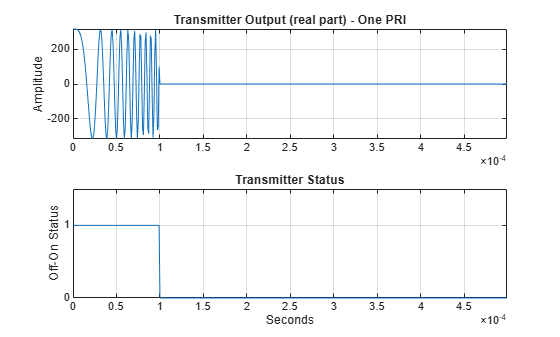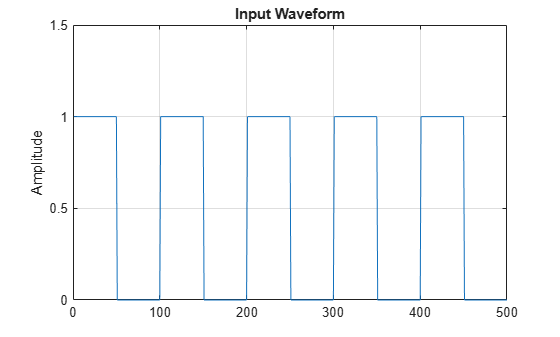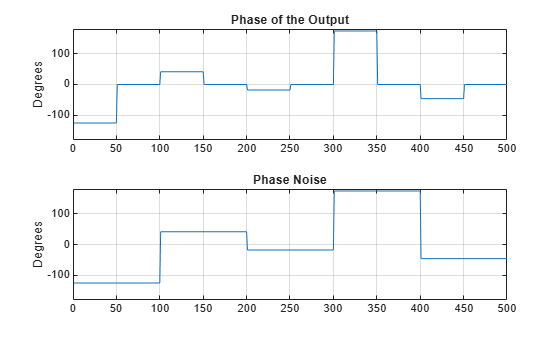## Transmitter

### Transmitter Object

The `phased.Transmitter` object lets you model key components of the radar equation including the peak transmit power, the transmit gain, and a system loss factor.

While the preceding functionality is important in applications dependent on amplitude such as signal detectability, Doppler processing depends on the phase of the complex envelope. In order to accurately estimate the radial velocity of moving targets, it is important that the radar operates in either a fully coherent or pseudo-coherent mode. In the fully coherent, or coherent on transmit, mode, the phase of the transmitted pulses is constant. Constant phase provides you with a reference to detect Doppler shifts.

A transmitter that applies a random phase to each pulse creates phase noise that can obscure Doppler shifts. If the components of the radar do not enable you to maintain constant phase, you can create a pseudo-coherent, or coherent on receive radar by keeping a record of the random phase errors introduced by the transmitter. The receiver can correct for these errors by modulation of the complex envelope. The `phased.Transmitter` object enables you to model both coherent on transmit and coherent on receive behavior.

The transmitter object has the following modifiable properties:

• `PeakPower` — Peak transmit power in watts

• `Gain` — Transmit gain in decibels

• `LossFactor` — Loss factor in decibels

• `InUseOutputPort` — Track transmitter's status. Setting this property to `true` outputs a vector of 1s and 0s indicating when transmitter is on and off. In a monostatic radar, the transmitter and receiver cannot operate simultaneously.

• `CoherentOnTransmit` — Preserve coherence among transmitter pulses. Setting this property to `true` (the default) models the operation of a fully coherent transmitter where the pulse-to-pulse phase is constant. Setting this property to `false` introduces random phase noise from pulse to pulse and models the operation of a non-coherent transmitter.

• `PhaseNoiseOutputPort` — Output the random pulse phases introduced by non-coherent operation of the transmitter. This property only applies if the `CoherentOnTransmit` property is `false`. By keeping a record of the random pulse phases, you can create a pseudo-coherent, or coherent on receive radar.

#### Transmit Linear FM Pulse

Amplify and transmit a linear FM pulse.

Note: This example runs only in R2016b or later. If you are using an earlier release, replace each call to the function with the equivalent `step` syntax. For example, replace `myObject(x)` with `step(myObject,x)`.

Construct a transmitter with a peak transmit power of 1000 watts, a transmit gain of 20 decibels (dB), and a loss factor of 0 dB. Set the `InUseOutPutPort` property to `true` to record the transmitter status. Pulse waveform values are scaled based on the peak transmit power and the ratio of the transmitter gain to loss factor.

```transmitter = phased.Transmitter('PeakPower',1e3,'Gain',20,... 'LossFactor',0,'InUseOutputPort',true)```
```transmitter = phased.Transmitter with properties: PeakPower: 1000 Gain: 20 LossFactor: 0 InUseOutputPort: true CoherentOnTransmit: true ```

The waveform scaling factor is `sqrt(PeakPower*db2pow(Gain - LossFactor))`

Construct a linear FM pulse waveform for transmission. Use a 100 μsec linear FM pulse having a bandwidth of 200 kHz. Use the default sweep direction and sample rate. Set the pulse repetition frequency (PRF) to 2 kHz. Obtain one pulse by setting the `NumPulses` property of the `phased.LinearFMWaveform` object to unity.

```waveform = phased.LinearFMWaveform('PulseWidth',100e-6,'PRF',2e3,... 'SweepBandwidth',200e3,'OutputFormat','Pulses','NumPulses',1);```

Generate the pulse by executing the `phased.LinearFMWaveform` waveform System object™. Then, transmit the pulse by executing the `phased.Transmitter` System object.

```wf = waveform(); [txoutput,txstatus] = transmitter(wf); t = unigrid(0,1/waveform.SampleRate,1/waveform.PRF,'[)'); subplot(211) plot(t,real(txoutput)) axis tight grid on ylabel('Amplitude') title('Transmitter Output (real part) - One PRI') subplot(212) plot(t,txstatus) axis([0 t(end) 0 1.5]) xlabel('Seconds') grid on ylabel('Off-On Status') set(gca,'ytick',[0 1]) title('Transmitter Status')```### Phase Noise

To model a coherent on receive radar, you can set the `CoherentOnTransmit` property to `false` and the `PhaseNoiseOutputPort` property to `true`. You can output the random phase added to each sample when you execute the System object™.

#### Transmit Rectangular Pulse with Phase Noise

This example illustrates adding phase noise to a rectangular pulse waveform having five pulses. A random phase is added to each sample of the waveform. Compute the phase of the output waveform and compare the phase to the phase noise returned when executing the System object™.

Note: This example runs only in R2016b or later. If you are using an earlier release, replace each call to the function with the equivalent `step` syntax. For example, replace `myObject(x)` with `step(myObject,x)`.

For convenience, set the gain of the transmitter to 0 dB, the peak power to 1 W, and seed the random number generator to ensure reproducible results.

```waveform = phased.RectangularWaveform('NumPulses',5); transmitter = phased.Transmitter('CoherentOnTransmit',false,... 'PhaseNoiseOutputPort',true,'Gain',0,'PeakPower',1,... 'SeedSource','Property','Seed',1000); wf = waveform(); [txtoutput,phnoise] = transmitter(wf); phdeg = rad2deg(phnoise); phdeg(phdeg>180)= phdeg(phdeg>180) - 360; plot(wf) title('Input Waveform') axis([0 length(wf) 0 1.5]) ylabel('Amplitude') grid on``````subplot(2,1,1) plot(rad2deg(atan2(imag(txtoutput),real(txtoutput)))) title('Phase of the Output') ylabel('Degrees') axis([0 length(wf) -180 180]) grid on subplot(2,1,2) plot(phdeg) title('Phase Noise'); ylabel('Degrees') axis([0 length(wf) -180 180]) grid on```The first figure shows the waveform. The phase of each pulse at the input to the transmitter is zero. In the second figure, the top plot shows the phase of the transmitter output waveform. The bottom plot shows the phase added to each sample. Focus on the first 100 samples. The pulse waveform is equal to 1 for samples 1-50 and 0 for samples 51-100. The added random phase is a constant -124.7° for samples 1-100, but this affects the output only when the pulse waveform is nonzero. In the output waveform, you see that the output waveform has a phase of -124.7° for samples 1-50 and 0 for samples 51-100. Examining the transmitter output and phase noise for samples where the input waveform is nonzero, you can see that the phase output the System object and the phase of the transmitter output agree.